# RF-to-Bits Solution Offers Precise Phase and Magnitude Data for Material Analysis

### Introduction

When analyzing materials in remote locations, where placing probes within the material is not viable, a high-frequency transceiver can provide a practical method for accurately quantifying the material’s volume fractions without the adverse effects of direct exposure to the material. Quadrature demodulators offer a novel, robust way to measure magnitude and phase shift in these applications. The receiver signal chain presented here uses the ADL5380 broadband quadrature demodulator, the ADA4940-2 ultralow power, low distortion, fully differential ADC driver, and the AD7903 dual, differential, 16-bit, 1-MSPS PulSAR® ADC to provide accurate data while ensuring safe, economical operation.

In the receiver shown in Figure 1, a continuous wave signal is sent from the transmit (Tx) antenna, through the material to be analyzed, to the receive (Rx) antenna. The received signal will be attenuated and phase shifted relative to the original transmitted signal. This amplitude change and phase shift can be used to determine the content of media.

Magnitude and phase shifts can be directly correlated to the transmittance and reflectance properties of an element, as shown in Figure 2. In the case of oil-gas-water flow, for example, the permittivity, loss, and dispersion is high for water, low for oil, and extremely low for gas.

The receiver subsystem shown in Figure 3 converts an RF signal to digital to accurately measure magnitude and phase. The signal chain comprises a quadrature demodulator, a dual differential amplifier, and a dual, differential SAR ADC. The key goals of this design are high-precision phase and magnitude measurements with wide dynamic range for high-frequency RF inputs.

A quadrature demodulator provides an in-phase (I) signal and a quadrature (Q) signal that is exactly 90° out of phase. The I and Q signals are vector quantities, so the amplitude and phase shift of the received signal can be calculated using trigonometric identities, as shown in Figure 4. The local-oscillator (LO) input is the original transmitted signal and the RF input is the received signal. The demodulator generates a sum and difference term. Both signals are at the exact same frequency, ωLO = ωRF, so the high-frequency sum term will get filtered, while the difference term resides at dc. The received signal will have a different phase, ϕRF, than that of the transmitted signal, ϕLO. This phase shift, ϕLO – ϕRF, is due to the permittivity of the media and will help define the material content.

A real-world I/Q demodulator has many imperfections, including quadrature phase error, gain imbalance, and LO-to-RF leakage, all of which could degrade the quality of the demodulated signal. To select a demodulator, first determine the requirements for RF input frequency range, amplitude accuracy, and phase accuracy.

Powered from a single 5-V supply, the ADL5380 accepts RF or IF input frequencies from 400 MHz to 6 GHz, making it ideal for the receiver signal chain. Configured to provide a 5.36-dB voltage conversion gain, its differential I and Q outputs can drive a 2.5-V p-p differential signal into a 500-Ω load. Its 10.9-dB NF, 11.6-dBm IP1dB, and 29.7-dBm IIP3 @ 900 MHz provide outstanding dynamic range, while its 0.07-dB amplitude balance and 0.2° phase balance achieve excellent demodulation accuracy. Manufactured using an advanced SiGe bipolar process, it is available in a tiny 4-mm × 4-mm, 24-lead LFCSP package.

The ADA4940-2 fully differential dual amplifier’s excellent dynamic performance and adjustable output common-mode make it ideal for driving high-resolution, dual SAR ADCs. Powered from a single 5-V supply, it provides ±5-V differential outputs with a 2.5-V common-mode. Configured to provide a gain of 2 (6 dB), it drives the ADC inputs to full scale. The RC filter (22 Ω/2.7 nF) helps to limit the noise and reduces the kickback coming from the capacitive DAC at the ADC input. Manufactured using a proprietary SiGe complementary bipolar process, it is available in a tiny 4-mm × 4-mm, 24-lead LFCSP package.

The AD7903 dual, 16-bit, 1-MSPS successive-approximation ADC offers excellent precision, with ±0.006% FS gain error and ±0.015-mV offset error. Operating from a single 2.5-V power supply, it dissipates only 12 mW at 1 MSPS. The main goal of using a high-resolution ADC is to achieve ±1° phase accuracy, especially when the input signal has a small dc magnitude. The 5-V reference required by the ADC is generated by the ADR435 low-noise reference.

As shown in Figure 5, the receiver subsystem is implemented using the ADL5380-EVALZ, EB-D24CP44-2Z, EVAL-AD7903SDZ, and EVAL-SDP-CB1Z evaluation kits. These circuit components are optimized for interconnection in the subsystem. Two high-frequency, phase-locked input sources provide the RF and LO input signals.

Table 1 summarizes the input and output voltage levels for each of the components in the receiver subsystem. An 11.6-dBm signal at the demodulator’s RF input will produce an input within –1 dB of the ADC’s full-scale range. The table assumes a 500-Ω load, 5.3573-dB conversion gain, and –4.643-dB power gain for the ADL5380, and 6-dB gain for the ADA4940-2. The calibration routine and performance results achieved for this receiver subsystem will be discussed in the following sections.

Table 1. Input and output voltage levels at each component in the receiver subsystem

 RF Input (dBm) ADL5380 Output AD7903 Input (dBFS) (dBm) (V p-p) +11.6 +6.957 4.455 –1.022 0 –4.643 1.172 –12.622 –20 –24.643 0.117 –32.622 –40 –44.643 0.012 –52.622 –68 –72.643 466µ –80.622

The receiver subsystem contains three major error sources: offset, gain, and phase.

The individual differential dc magnitudes of the I and Q channels have sinusoidal relationships with respect to the relative phase of the RF and LO signals. As a result, the ideal dc magnitude of the I and Q channels can be calculated as follows:(3)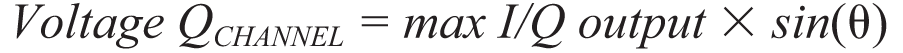(4)

As the phase moves through the polar grid, some locations should ideally produce the same voltage. For example, the voltage on the I (cosine) channel should be identical with phase shifts of +90° or –90°. However, a constant phase shift error, independent of the relative phase of RF and LO, will cause the subsystem channel to generate different results for input phases that should produce the same dc magnitude. This is illustrated in Figure 6 and Figure 7, where two different output codes are generated when the input should be at 0 V. In this case, the –37° phase shift is much larger than expected in a real-world system containing phase-locked loops. The result is +90° actually appearing as +53° and –90° as –127°.

Results were gathered in 10° steps from –180° to +180°, with the uncorrected data generating the elliptical shapes shown in Figure 6 and Figure 7. This error can be accounted for by determining the amount of additional phase shift present in the system. Table 2 shows that the system phase shift error is constant throughout the transfer function.

Table 2. Summary of receiver subsystems measured phase shift for 0-dBm RF input amplitude.

 Input Phase RF to LO Average I Ch Output Code Average Q Ch Output Code I Ch Voltage Q Ch Voltage Measured Phase Measured Receiver Subsystem Phase Shift –180° –5851.294 +4524.038 –0.893 +0.690 +142.29° –37.71° –90° –4471.731 –5842.293 –0.682 –0.891 –127.43° –37.43° 0° +5909.982 –4396.769 +0.902 –0.671 –36.65° –36.65° +90° +4470.072 +5858.444 +0.682 +0.894 +52.66° –37.34° +180° –5924.423 +4429.286 –0.904 +0.676 +143.22° –36.78°

### System Phase Error Calibration

With a step size of 10°, the average measured phase shift error was –37.32° for the system shown in Figure 5. With this additional phase shift known, the adjusted subsystem dc voltages can now be calculated. The variable ϕPHASE_SHIFT is deﬁned as the average observed additional system phase shift. The dc voltage generated in the phase-compensated signal chain can be computed as: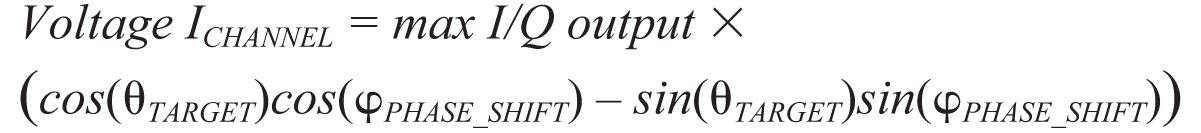(5)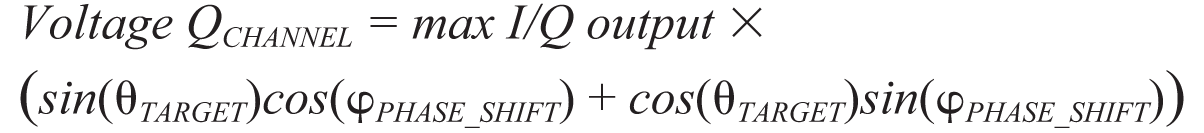(6)

Equation 5 and Equation 6 provide the target input voltage for a given phase setting. The subsystem has now been linearized and the offset error and gain error can now be corrected. The linearized I and Q channel results can also be seen in Figure 6 and Figure 7. A linear regression on the data sets generates the best ﬁt line shown in the ﬁgures. This line is the measured subsystem transfer function for each conversion signal chain.

### System Offset Error and Gain Error Calibration

The offset of each signal chain within the receiver subsystem should ideally be 0 LSB, but the measured offsets were –12.546 LSB and +22.599 LSB for I and Q channels, respectively. The slope of the best fit line represents the slope of the subsystem. The ideal subsystem slope can be calculated as: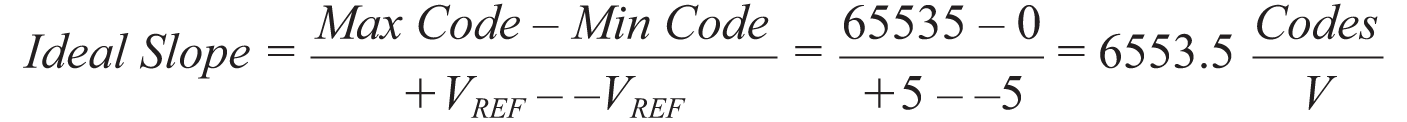(7)

The results in Figure 6 and Figure 7 show that that measured slopes were 6315.5 and 6273.1 for the I and Q channels, respectively. These slopes must be adjusted to correct the system gain error. Correcting for gain error and offset error ensures that the signal magnitude computed using Equation 1 matches the ideal signal magnitude. The offset correction is just the opposite of the measured offset error:(8)

The gain error correction coefficient is: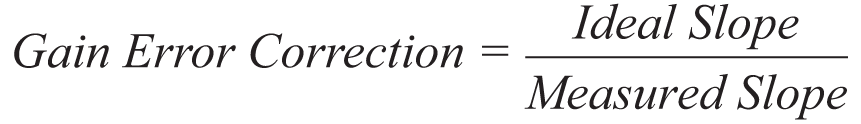(9)

The received conversion result can be corrected by:(10)

The subsystem’s calibrated dc input voltage is calculated as:(11)

Equation 11 should be used on both I and Q channels to compute the perceived analog input voltage for each subsystem signal chain. These fully adjusted I and Q channel voltages are used to compute the RF signal amplitude as defined by the individual dc signal magnitudes. To evaluate the accuracy of the full calibration routine, the collected results can be converted to ideal subsystem voltages produced at the output of the demodulator as if no phase shift error was present. This can be done by multiplying the average dc magnitude computed previously by the sinusoidal fraction of the measured phase at each trial with the computed phase shift error removed. The calculation appears as follows:(12)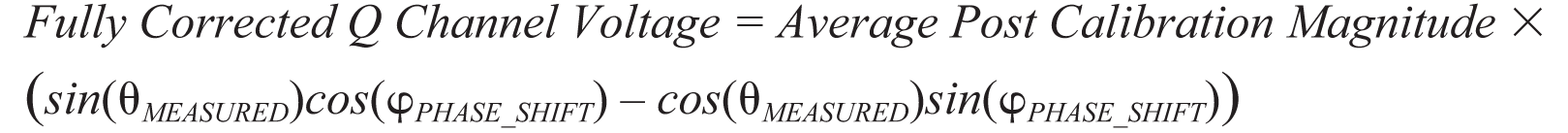(13)

The ϕPHASE_SHIFT is the phase error previously computed and the average post calibration magnitude is the dc magnitude result from Equation 1 that has been compensated for offset error and gain error. Table 3 shows the results of the calibration routine at various target phase inputs for the 0 dBm RF input amplitude case. The calculations performed in Equation 12 and Equation 13 are the correction factors to be built into any system intended to sense phase and magnitude in the manner presented here.

Table 3. Results achieved at certain target phase inputs with 0 dBm RF input amplitude.

 Target Phase I Ch Fully Corrected Input Voltage Q Ch Fully Corrected Input Voltage Fully Corrected Phase Result Absolute Measured Phase Error –180° –1.172 V +0.00789 V –180.386° 0.386° –90° –0.00218 V –1.172 V –90.107° 0.107° 0° +1.172 V +0.0138 V +0.677° 0.676° 0.676° +0.000409 V +1.171 V +89.98° 0.020° +180° –1.172 V +0.0111 V +180.542° 0.541°

Figure 8 is a histogram of the measured absolute phase error showing better than 1° accuracy for every 10° step from –180° to +180°.Figure 8. Measured absolute phase error histogram for 0-dBm input level with 10° phase steps.

For accurate phase measurements at any given input level, the perceived phase shift error (ϕPHASE_SHIFT) of RF relative to LO should be constant. If the measured phase shift error begins to change as a function of the target phase step (ϕTARGET) or amplitude, then the calibration routine presented here will begin to lose accuracy. Evaluation results at a room temperature show that the phase-shift error is relatively constant for RF amplitudes ranging from a maximum of 11.6 dBm to approximately –20 dBm at 900 MHz.

Figure 9 demonstrates the dynamic range of the receiver subsystem along with the corresponding amplitude induced additional phase error. As the input amplitude decreases past –20 dBm, the phase error calibration accuracy begins to degrade. The system user will need to determine the accept-able level of signal chain error to determine the minimum acceptable signal magnitude.

The results presented in Figure 9 were collected with a 5-V ADC reference. The magnitude of the ADC reference can be reduced, providing a smaller quantization level for the system. This will provide an incremental improvement in phase error accuracy for small signals, but increases the chance for system saturation. To increase system dynamic range, another attractive option is implementing an oversampling scheme that will increase the noise-free-bit resolution of the ADC. Every doubling in samples averaged will provide a ½ LSB increase in system resolution. The oversampling ratio for a given resolution increase is calculated as follows:(14)

Oversampling will reach a point of diminishing returns when the noise amplitude is no longer sufficient to randomly change the ADC output code from sample to sample. At this point, the effective resolution of the system can no longer be increased. The bandwidth reduction from oversampling is not a significant concern because the system is measuring signals with a slowly changing magnitude.

The AD7903 evaluation software is available with a calibration routine that allows the user to correct the ADC output results for the three sources of error: phase, gain, and offset. The user will need to collect uncorrected results with their system to determine the calibration coefficients calculated in this article. Figure 10 shows the GUI with the calibration coefficients highlighted. Once the coefficients are determined, this panel can also be used to deliver phase and magnitude results from the demodulator. The polar plot provides a visual indication of the observed RF input signal. The amplitude and phase calculations are performed using Equation 1 and Equation 2. The oversampling ratio can be controlled by adjusting the number of samples per capture using the “Num Samples” drop-down box.